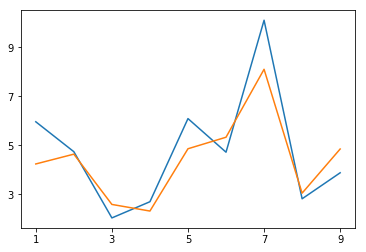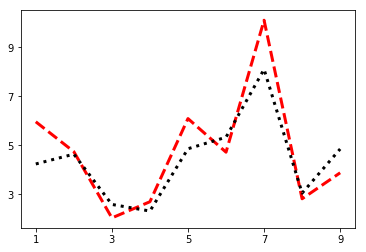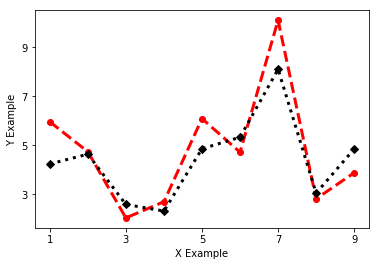# Data Visualization in Python

In this post, we will look at how to set up various features of a graph in Python. The fine tune tweaks that can be made when creating a data visualization can be enhanced the communication of results with an audience. This will all be done using the matplotlib module available for python. Our objectives are as follows

• Make a graph with  two lines
• Set the tick marks
• Change the linewidth
• Change the line color
• Change the shape of the line
• Add a label to each axes
• Annotate the graph
• Add a legend and titleWe will use two variables from the “toothpaste” dataset from the pydataset module for this demonstration. Below is some initial code.

```from pydataset import data
import matplotlib.pyplot as plt
DF = data('toothpaste')```

Make Graph with Two Lines

To make a plot you use the .plot() function. Inside the parentheses you out the dataframe and variable you want. If you want more than one line or graph you use the .plot() function several times. Below is the code for making a graph with two line plots using variables from the toothpaste dataset.

```plt.plot(DF['meanA'])
plt.plot(DF['sdA'])```To get the graph above you must run both lines of code simultaneously. Otherwise, you will get two separate graphs.

Set Tick Marks

Setting the tick marks requires the use of the .axes() function. However, it is common to save this function in a variable called axes as a handle. This makes coding easier. Once this is done you can use the .set_xticks() function for the x-axes and .set_yticks() for the y axes. In our example below, we are setting the tick marks for the odd numbers only. Below is the code.

```ax=plt.axes()
ax.set_xticks([1,3,5,7,9])
ax.set_yticks([1,3,5,7,9])
plt.plot(DF['meanA'])
plt.plot(DF['sdA'])```Changing the Line Type

It is also possible to change the line type and width. There are several options for the line type. The important thing here is to put this information after the data you want to plot inside the code. Line width is changed with an argument that has the same name. Below is the code and visual

```ax=plt.axes()
ax.set_xticks([1,3,5,7,9])
ax.set_yticks([1,3,5,7,9])
plt.plot(DF['meanA'],'--',linewidth=3)
plt.plot(DF['sdA'],':',linewidth=3)```Changing the Line Color

It is also possible to change the line color. There are several options available. The important thing is that the argument for the line color goes inside the same parentheses as the line type. Below is the code. r means red and k means black.

```ax=plt.axes()
ax.set_xticks([1,3,5,7,9])
ax.set_yticks([1,3,5,7,9])
plt.plot(DF['meanA'],'r--',linewidth=3)
plt.plot(DF['sdA'],'k:',linewidth=3)```Change the Point Type

Changing the point type requires more code inside the same quotation marks where the line color and line type are. Again there are several choices here. The code is below

```ax=plt.axes()
ax.set_xticks([1,3,5,7,9])
ax.set_yticks([1,3,5,7,9])
plt.plot(DF['meanA'],'or--',linewidth=3)
plt.plot(DF['sdA'],'Dk:',linewidth=3)```Adding LAbels is simple. You just use the .xlabel() function or .ylabel() function. Inside the parentheses, you put the text you want in quotation marks. Below is the code.

```ax=plt.axes()
ax.set_xticks([1,3,5,7,9])
ax.set_yticks([1,3,5,7,9])
plt.xlabel('X Example')
plt.ylabel('Y Example')
plt.plot(DF['meanA'],'or--',linewidth=3)
plt.plot(DF['sdA'],'Dk:',linewidth=3)```Annotation allows you to write text directly inside the plot wherever you want. This involves the use of the .annotate function. Inside this function, you must indicate the location of the text and the actual text you want added to the plot. For our example, we will add the word ‘python’ to the plot for fun.

The .legend() function allows you to give a description of the line types that you have included. Lastly, the .title() function allows you to add a title. Below is the code.

```ax=plt.axes()
ax.set_xticks([1,3,5,7,9])
ax.set_yticks([1,3,5,7,9])
plt.xlabel('X Example')
plt.ylabel('Y Example')
plt.annotate(xy=[3,4],text='Python')
plt.plot(DF['meanA'],'or--',linewidth=3)
plt.plot(DF['sdA'],'Dk:',linewidth=3)
plt.legend(['1st','2nd'])
plt.title("Plot Example")```Conclusion

Now you have a practical understanding of how you can communicate information visually with matplotlib in python. This is barely scratching the surface in terms of the potential that is available.